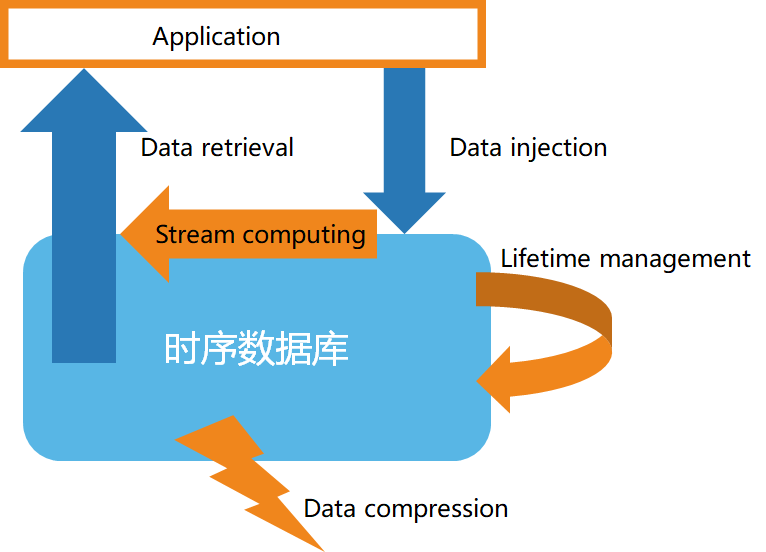# 時序數據庫的流計算支持

• 歷史數據的不變性
• 數據的有效性
• 數據的時效性
• 結構化的數據
• 數據的大量性• 高速的數據入庫
• 數據的生命週期管理
• 數據的流處理
• 高效的數據查詢
• 定製的數據壓縮

1）過濾和轉換 （filter & map）

2）聚合以及窗口函數 （reduce，aggregation/window）

3）多數據流合併以及模式匹配 （joining & pattern detection）

4）從流到塊處理

• 案例一：使用定製化的流計算 API，如下面例子所示：

``````from(bucket: "mydb")
|> range(start: -1h)
|> filter(fn: (r) => r["_measurement"] == "mymeasurement")
|> map(fn: (r) => ({ r with value: r.value * 2 }))
|> filter(fn: (r) => r.value > 100)
|> aggregateWindow(every: 1m, fn: sum, createEmpty: false)
|> group(columns: ["location"])
|> join(tables: {stream1: {bucket: "mydb", measurement: "stream1", start: -1h}, stream2: {bucket: "mydb", measurement: "stream2", start: -1h}}, on: ["location"])
|> alert(name: "value_above_threshold", message: "Value is above threshold", crit: (r) => r.value > 100)
|> to(bucket: "mydb", measurement: "output", tagColumns: ["location"])``````

• 案例二：使用類 SQL 指令，創建流計算以及定義流計算規則，如下：

``````CREATE STREAM current_stream
TRIGGER AT_ONCE
INTO current_stream_output_stb AS
SELECT
_wstart as start,
_wend as end,
max(current) as max_current
FROM meters
WHERE voltage <= 220
INTEVAL (5S) SLIDING (1s);``````